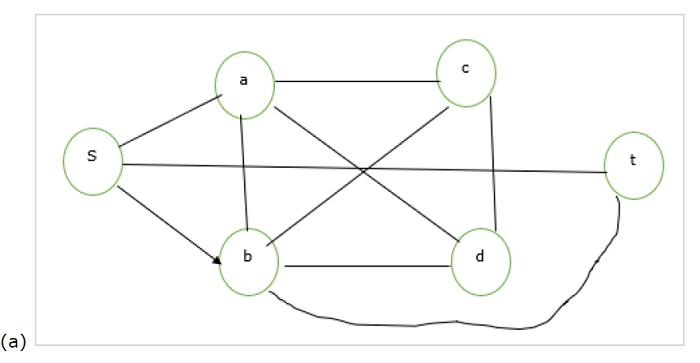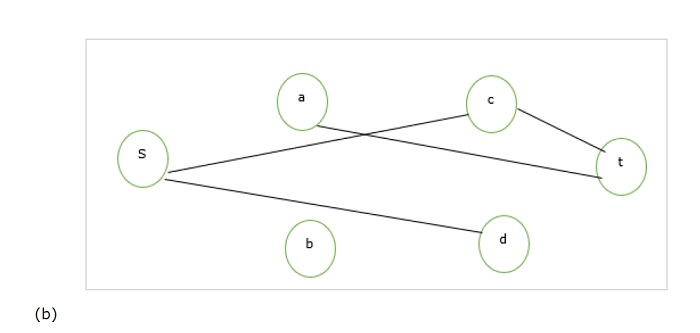# Prove that the vertex cover is NP complete in TOC

It is the subset(minimum size) of vertices of a graph G such that every edge in G incident to at least one vertex in G.

## Vertex Cover (VC) Problem

To prove VC is NP-complete we have to prove the following −

• VC is Non-deterministic Polynomial (NP).

• A NPC problem can be reduced into VC.

To prove VC is NP, find a verifier which is a subset of vertices which is VC and that can be verified in polynomial time. For a graph of n vertices it can be proved in O(n2). Thus, VC is NP.

Now consider the “clique” problem which is NPC and reduce it into VC to prove NPC. Clique of a graph G is a subset of vertices such that these vertices form a complete subgraph in the given graph G.

The two graphs titles (a) and (b) are given below for the VC problem −Consider graph (a), here clique is {a,b,c,d}.

Now calculate a graph as shown in (b) which is as follows −

Completed graph of all the vertices in (a) – (a)

For the graph (b), we can say that the vertex cover is {s,t} which covers all the edges of the (b). This {s,t} = {a,b,c,d,s,t} – {Clique of (a)} Thus conversely, we can say that we can reduce clique into the VC problem and conversely can find both VC and clique of a given undirected graph. This means VC is NP-complete reducible.

Hence proved that VC is an NPC.